Home Maths Main Page

A fun addition exercise Maths worksheet for grade 1 (first grade) students and kids with rabbit and canvas theme. Write the solutions and color the rabbit. (Vertical Single Digit Addition Worksheet for kids)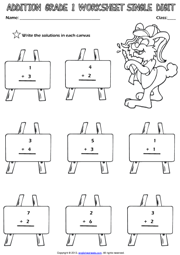A practical single digit picture addition exercise maths worksheet for grade 1 (first grade) students and kids with animals theme. Count the animals. Add them and then write the solutions.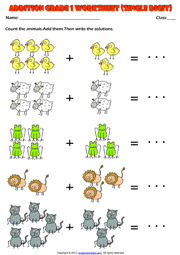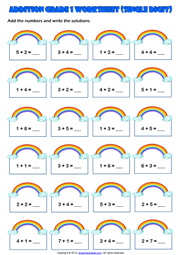##### Count and Add Single Digit Maths Exercise Worksheet
Count the fruits, fill in the blanks with their numbers and add them. An addition exercises worksheet with fruit theme for grade 1 (first grade) students and kids. (Horizontal Addition Worksheet for first grade students)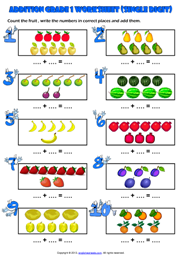A colorful single digit addition exercise Maths worksheet for grade 1 (first grade) students and kids with lovely dogs theme. Add the numbers and write the solutions. (Vertical Addition Worksheet for kids)## Addition Exercise Maths Worksheet With Two Digit Results

A fun addition exercise maths worksheet for grade 1 (first grade) students and kids with one digit and two digits numbers and robots theme. Add the numbers and write the solutions. (Vertical Addition Worksheet with two digit results for kids)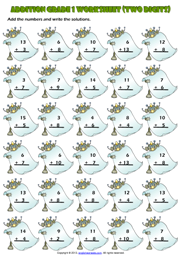### Two Digits Addition Maths Worksheet Without Carrying

A colorful addition exercise maths worksheet without carrying for grade 1 (first grade) students and kids with two digit numbers and ships and sailor theme. Add the numbers and write the solutions. (Vertical Addition Worksheet for kids)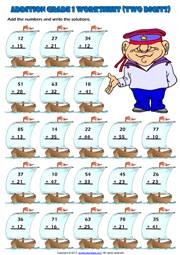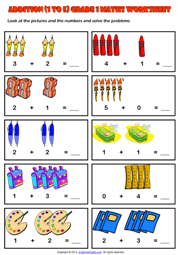Next Topic Maths Main Page Home